﻿ 20-sim webhelp > Toolboxes > Control Toolbox > Controller Design Editor > Controller Design Editor

# Controller Design Editor

The 20-sim Controller Design Editor allows you to enter or edit linear time-invariant models of a plant with control loop. The control loop is separated in a compensator, prefilter and measurement. You can open the Controller Design Editor from the Tools menu of the 20-sim Editor or by clicking Go Down on a ControlledLinearSystem model that was inserted in the Editor.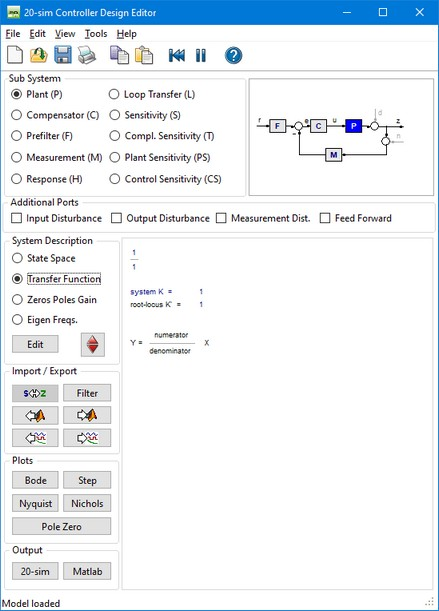## Sub System

 • You can choose to show a single element in the loop:
 • Plant (P), Compensator (C), Prefilter (F) or Measurement (M)
 • or view the response of the system:
 • Response (H), Loop Transfer (L), Sensitivity (S), Compl. Sensitivity (T), Plant Sensitivity (PS) or Control Sensititvity (CS).

The corresponding transfer function is show in the editor.

Select one of the radio buttons Input Disturbance, Output Disturbance, Measurement Dist. or Feedforward. The first three options allow you to use external disturbance in your model. The last option allows you to use an external feedforward controller (e.g. a B-Spline network). The selected inputs are shown in the picture.

## System Description

 • Pressing the Edit button will open a dialog for editing the linear system. Each description (State Space, Transfer Function, Zeros Poles Gain or Eigen Frequency) has a special dialog and can be used to specify continuous-time and discrete-time systems.
 • In the 20-sim Simulator, out of an existing (non-linear) model a symbolic linear-system can be derived by means of linearization. This means that the relevant model parameters are preserved in the Linear System and the parameters button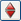can be used to change parameters.

## Import/Export

 • Pressing the s<->z  button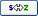will transform a continuous-time linear system into a discrete-time linear system and back.
 • Pressing the Filter button opens the Filter Editor, where a filter can be designed. This filter can then be combined with the current linear system or replace the current linear system. If the linear system is a discrete-time system, the designed analog filter is automatically transferred into its digital equivalent.
 • Pressing the From Matlab button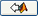and the To Matlab button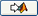allows for an instant exchange of the linear system with the Matlab workspace. Information is transferred numerically (no parameter relations are preserved)
 • The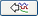button is only active when a Linear System has been imported through linearization. Clicking the button will import parameters from the simulation.
 • The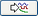button is only active when a Linear System has been imported through linearization. Clicking the button will export the current parameters to the simulation.

## Plots

You can inspect the time- and frequency responses of the selected transfer function using:

 • Step Response
 • Bode Plot
 • Nyquist Plot
 • Nichols Chart
 • Poles and Zeros (including root locus)

## Output

 • Clicking the 20-sim button will export the linear system as a new 20-sim submodel.
 • Clicking the Matlab button will export the 20-sim Linear System to a Matlab m-file. If the Linear System is symbolic, all parameter relations are preserved.